# High School Physics : Understanding Motion in One Dimension

## Example Questions

1 2 4 Next →

### Example Question #31 : Motion And Mechanics

A drone needs to reach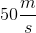to take off and it takes 600m to reach this speed. If the drone's acceleration is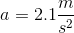, how many seconds does it take to reach takeoff speed?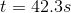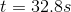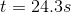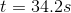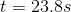Explanation:

We know the final velocity, that initial velocity is 0, and the acceleration of the drone. We can find the time it takes to reach its takeoff speed by using the following kinematics formula: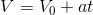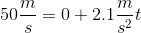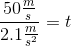### Example Question #32 : Motion And Mechanics

A tennis ball is thrown straight up and it is caught at the same height the person released the ball from their hand. Which of the following is false? Ignore air resistance.

The velocity will change sign at the the top of the motion

The time the tennis ball is traveling up is equal to the time it falls down

The speed of the tennis ball as it leaves the person's hand is the same as when it is caught

Acceleration and velocity point in the same direction the entire time

All of these answers are true

Acceleration and velocity point in the same direction the entire time

Explanation:

The question asks to point out the false statement. Everything on Earth is accelerated downwards by gravity, all the time, by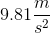. Think of gravity as having a negative sign. When the ball is thrown up, acceleration is working against the velocity slowing the ball down. Their signs are opposite. But when the ball is falling back down to Earth, the velocity and acceleration have the same sign. So velocity and acceleration will not always have the same sign.

If gravity could be said to have a negative sign since it pulls everything downward, then an upward velocity would have a positive (and opposing sign). At the top of the trajectory when the ball's upward velocity is finally overcome by gravity, the sign of the velocity becomes negative as it now points back down to Earth. So it is true velocity changes sign at the top.

Since the ball is caught at the same height, both the time up and time down are equal and it will be traveling at the same speed. Since gravity is the only force acting on it, the ball loses all its velocity on the way up and regains that exact amount by the time it reaches the height it started the journey. This also makes the time up equal to the time it falls. If the same force acts with same strength (gravity) the entire time, why would either of these change?

### Example Question #33 : Motion And Mechanics

If you dropped a penny from the roof of a building 15m high, how much time would elapse before the penny hits the ground?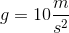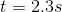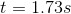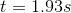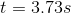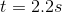Explanation:

Use one of the kinematic equations. The best way to select the correct one is by process of elimination based on which variables we have or don't. The problem gives us vertical displacement, gravity, and if we look closely, initial velocity (since the stone was "dropped" it equals 0). Based on these we will definitely use one that factors in displacement: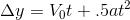Note that these kinematic equations normally have an x-variable for displacement, but since our problem has an object falling vertically we use y-variables for consistency.

Plug in given values and solve: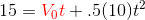The red term becomes 0 since the initial velocity is 0.

Simplify and solve for t: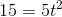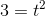### Example Question #34 : Motion And Mechanics

You are driving at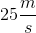when you slam on the brakes and skid to a halt just before the red light over the course of 2s. How far did the car slide? Assume uniform acceleration.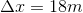None of these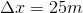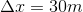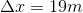Explanation:

We know the initial velocity, the final velocity, and the time it took for the car to stop moving after the brakes were applied. Given the final and initial velocity we can calculate average velocity. Average velocity multiplied by time is equal to the distance traveled.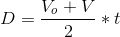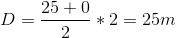### Example Question #35 : Motion And Mechanics

A flea can jump nearly 100 times its height. If a flea can jump 0.18m vertically, what is its take off speed (speed at which it leaves the ground)?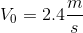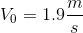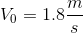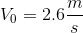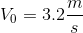Explanation:

Since we are given the vertical displacement, acceleration due to gravity, and we know that the final velocity in the y-direction is zero since it will be at the top of its jump. Use the following kinematics formula and solve for the initial velocity.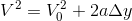Plug in known values and solve for initial velocity.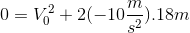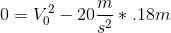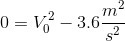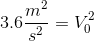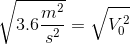### Example Question #36 : Motion And Mechanics

If you threw a tomato upwards with an initial velocity of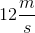, at what time(in seconds) would the tomato hit the ground?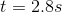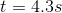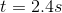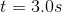Explanation:

Gravity accelerates everything downward by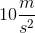. When the tomato is thrown upward with some velocity, gravity immediately begins to slowly reduce this velocity since the acceleration opposes the direction of the velocity.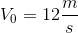(initial velocity)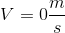(final velocity is equal to 0)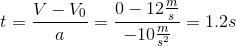In 1.2s the tomato will reach its maximum height. If you have ever thrown a ball upward you may have noticed how it appears to stop at the peak. We have just calculated the time it takes for that ball to appear to stop for a very small time and fall back down. Since our tomato must travel back to Earth, we double the time for its up and down motion (since they equal each other) to get 2.4s as the final answer.

1 2 4 Next →